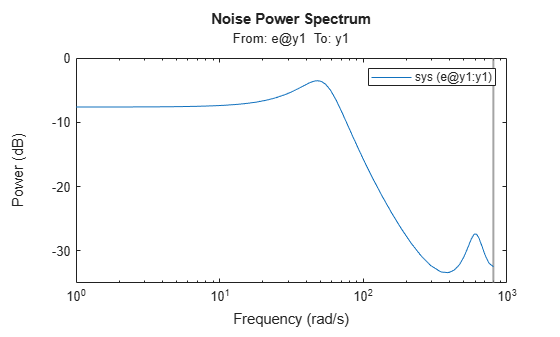# spectrum

Plot or return output power spectrum of time series model or disturbance spectrum of linear input/output model

## Syntax

``spectrum(sys)``
``spectrum(sys,{wmin, wmax})``
``spectrum(sys,w)``
``spectrum(sys1,...,sysN,w)``
``ps = spectrum(sys,w)``
``[ps,wout] = spectrum(sys)``
``````[ps,wout,sdps] = spectrum(sys)``````

## Description

### Plot Results

example

````spectrum(sys)` plots the output power spectrum of an identified time series model `sys` or the disturbance spectrum of an identified input/output model `sys`. The function chooses the frequency range and number of points automatically. If `sys` is a time series model, then `sys` represents the system:$y\left(t\right)=He\left(t\right)$Here, e(t) is Gaussian white noise and y(t) is the observed output.`spectrum` plots |H'H|, scaled by the variance of e(t) and the sample time.If `sys` is an input/output model, `sys` represents the system:$y\left(t\right)=Gu\left(t\right)+He\left(t\right)$Here, u(t) is the measured input, e(t) is Gaussian white noise, and y(t) is the observed output.In this case, `spectrum` plots the spectrum of the disturbance component He(t).For discrete-time models with sample time Ts, `spectrum` uses the transformation $z={e}^{j\omega {T}_{s}}$ to map the unit circle to the real frequency axis. The function plots the spectrum only for frequencies smaller than the Nyquist frequency π/Ts, and uses the default value of 1 time unit when `Ts` is unspecified.```

example

````spectrum(sys,{wmin, wmax})` creates a spectrum plot for frequencies ranging from `wmin` to `wmax`.```
````spectrum(sys,w)` creates a spectrum plot using the frequencies specified in the vector `w`.```

example

````spectrum(sys1,...,sysN,w)` creates a spectrum plot of several identified models on a single plot. The `w` argument is optional.You can specify a color, line style, and marker for each model. For example, `spectrum(sys1,'r',sys2,'y--',sys3,'gx')` uses red for `sys1`, yellow dash markers for `sys2`, and green `x` markers for `sys3`.```

### Return Results

````ps = spectrum(sys,w)` returns the power spectrum amplitude of `sys` for the specified frequencies `w`. This syntax does not plot the spectrum.```
````[ps,wout] = spectrum(sys)` returns the frequency vector `wout` for which the output power spectrum is computed.```
``````[ps,wout,sdps] = spectrum(sys)``` returns the estimated standard deviation of the power spectrum.```

## Examples

collapse all

`load iddata9 z9`

Estimate a fourth-order AR model using a least-squares approach.

`sys = ar(z9,4,'ls');`

Plot the output spectrum of the model.

`spectrum(sys);`To change display options in the plot, right-click the plot to access the context menu. For example:

• To view the confidence region for the simulated response, select Characteristics > Confidence Region.

• To specify a number of standard deviations to plot, select Properties. Then, in the property editor, select the Options tab and specify the number of standard deviations in Number of standard deviations for display. The default value is 1 standard deviation.

`load iddata1 z1;`

Estimate a single-input single-output state-space model.

`sys = n4sid(z1,2);`

Plot the noise spectrum for the model. Specify a frequency range from 0.1 to 50 rad/sec.

`spectrum(sys,{0.1,50});`The function plots the spectrum, but limits the frequency range to the Nyquist frequency of approximately 31.4 rad/s.

Create an input consisting of the sum of five sinusoids, each spread over the full frequency range. Compare the spectrum of this signal with that of its square.

Create a sum-of-sinusoids input that extends for 20 periods, with each period containing 100 samples. Specify that the signal combine 5 sinusoids of random phase, using 10 trials to find the set with the lowest signal spread. For more information on this step, see `idinput`.

`u = idinput([100 1 20],'sine',[],[],[5 10 1]);`

Create an input-only `iddata` object `u` that contains the input `u` and has a period of 100.

`u = iddata([],u,1,'per',100);`

Square the input values and store them in new `iddata` object `u2`.

```u2 = u.u.^2; u2 = iddata([],u2,1,'per',100);```

Use `etfe` to estimate empirical transfer function models from `u` and `u2`. Plot the power spectra of these models together. Use different marker colors and types to distinguish the spectrum sources.

`spectrum(etfe(u),'r*',etfe(u2),'+')`The plot shows some frequency splitting where the `u2`-based spectrum does not line up with the `u`-based spectrum, but instead contains two spectral points that flank certain `u`-based points. This splitting indicates the nonlinearity of the squared system.

## Input Arguments

collapse all

Identified model, specified as an `idpoly` object, an `idproc` object, an `idss` object, or an `idtf` object.

• If `sys` is a time series model, then `sys` represents the system:

`$y\left(t\right)=He\left(t\right)$`

Here, e(t) is Gaussian white noise and y(t) is the observed output.

• If `sys` is an input/output model, then `sys` represents the system:

`$y\left(t\right)=Gu\left(t\right)+He\left(t\right)$`

Here, u(t) is the measured input, e(t) is Gaussian white noise, and y(t) is the observed output.

Minimum frequency of the frequency range for which to plot the spectrum, specified as a positive number.

Specify `wmin` in rad/`TimeUnit`, where `TimeUnit` is `sys.TimeUnit`.

For an example of specifying `wmin`, see Plot Noise Spectrum of SISO Linear Identified Model.

Maximum frequency of the frequency range for which to plot the spectrum, specified as a positive number. By default, the function uses the Nyquist frequency of `sys` as `wmax`.

Specify `wmax` in rad/`TimeUnit`, where `TimeUnit` is `sys.TimeUnit`. If you specify `wmax` to be greater than the Nyquist frequency, then `spectrum` uses the Nyquist frequency instead.

For an example of specifying `wmax`, see Plot Noise Spectrum of SISO Linear Identified Model.

Frequencies for which to plot the spectrum, specified as a vector of positive numbers.

Specify `w` in `rad/TimeUnit`, where `TimeUnit` is `sys.TimeUnit`.

## Output Arguments

collapse all

Power spectrum amplitude, returned as a numeric vector or a numeric array.

• For single-output models, `ps` is a 1-by-1- Nw array, where Nw is the length of the frequency vector.

• For multiple-output models, `ps` is an Ny-by-Ny-by-Nw array, where Ny is the number of outputs. `ps(:,:,k)` corresponds to the power spectrum for the frequency at `w(k)`.

For amplitude values in dB, type ```psdb = 10*log10(ps)```.

Frequencies for which the spectrum is plotted, returned as a numeric vector in units of rad/`TimeUnit`, where `TimeUnit` is `sys.TimeUnit`. If you supply `w` as an input argument, the function returns the identical vector in `wout`.

Estimated standard deviation of the power spectrum, returned as an array with the same dimensions as `ps`.

## Version History

Introduced in R2012a